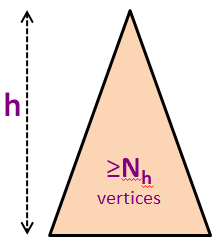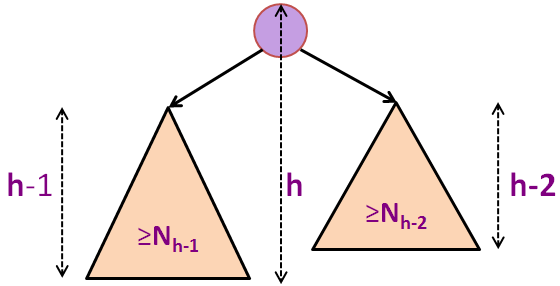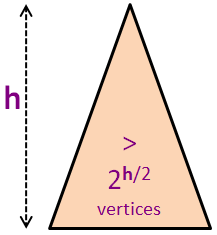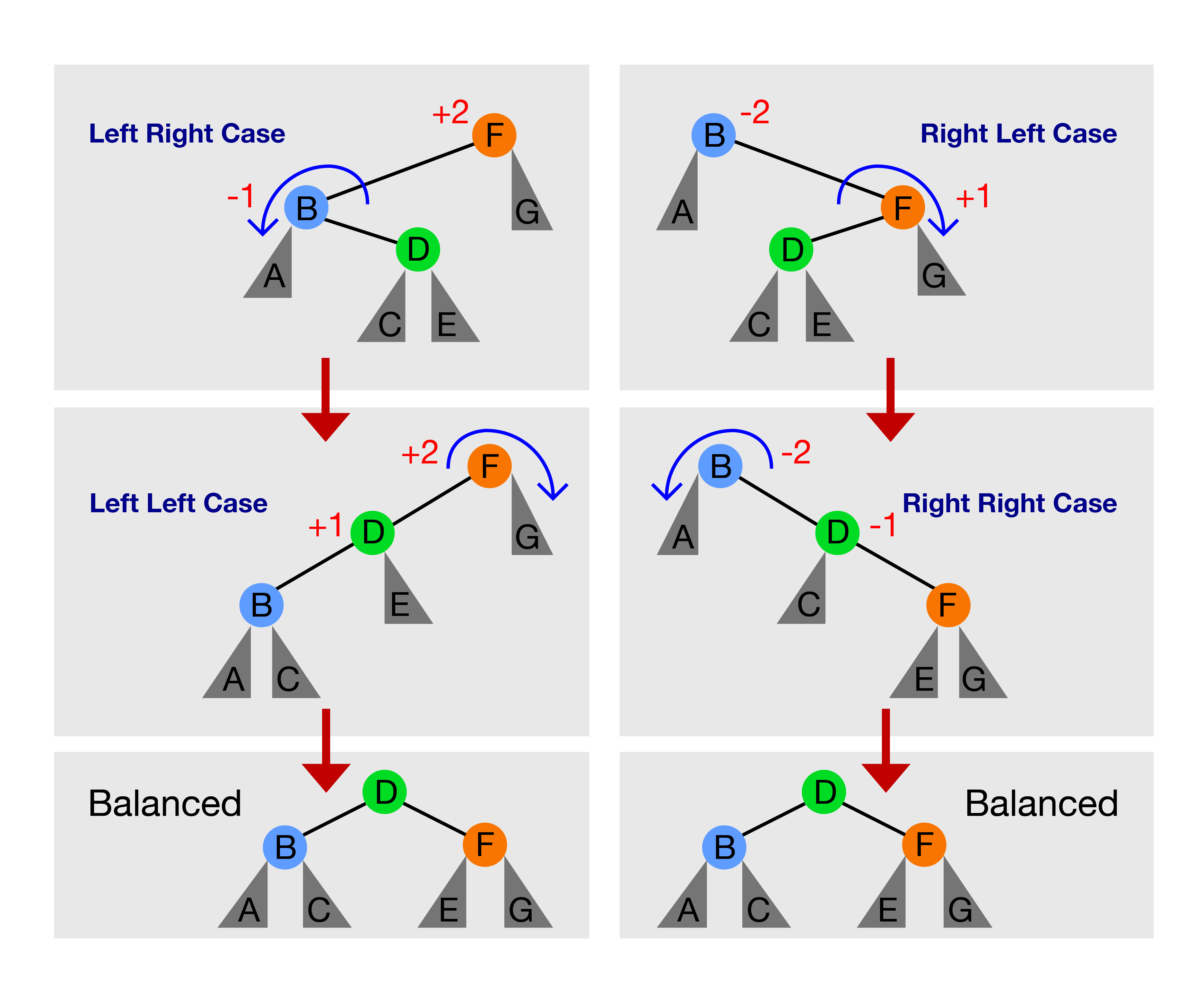# 二叉搜索树

## 3. 动机

1. 搜索（v） - 确定 ADT 中是否存在 v，
2. 插入（v） - 将 v 插入ADT，
3. 删除（v） - 从 ADT 中删除 v 。

### 3-2. 使用Sorted Array / Vector

2. 插入（v）可以在O(1)中实现，只需将v放在数组的后面，
3. 删除（v）也在O(N)中运行，因为我们必须首先搜索已经O(N)的v，然后用 O(N) 时间删除后处理被删除元素的空位。

### 3-3. 使用Sorted Array / Vector

1. 搜索（v）现在可以在O(log N),中实现，因为我们现在可以在已排序的数组上使用二进制搜索，
2. 插入（v）现在在O(N)中运行，因为我们需要实现类似插入的策略以使数组保持排序状态，
3. 删除（v）在O(N)中运行，因为即使 Search(v) O(log N)中运行，我们仍然需要在删除后关闭间隙 - 在O（N）中。

### 3-4. O（log N）复杂性？

 N ≈ 1 000 ≈ 1 000 000 ≈ 1 000 000 000 log N 10 Only 20 :O Only 30 :O:O

1. 找到 Min()/ Max() 元素，
2. 找到 Successor(v) - '下一个更大' / Predecessor(v) - '上一个更小'元素，
3. 按排序顺序列出元素，
4. X＆Y操作 - 因 NUS 教学目的被隐藏
5. 还有其他可能的操作。

### 3-6. 答案

[This is a hidden slide]

### 3-7. 链表怎么样？

Quiz: Can we perform all basic three Table ADT operations: Search(v)/Insert(v)/Remove(v) efficiently (read: faster than O(N)) using Linked List?

Yes
No

### 3-8. 答案

[This is a hidden slide]

### 3-9. 哈希表 (Hash Table) 怎么样？

Quiz: So what is the point of learning this BST module if Hash Table can do the crucial Table ADT operations in unlikely-to-be-beaten expected O(1) time?

There are valid reasons, which are ____
There is no point, so this BST module can be ignored

### 3-10. 答案

[This is a hidden slide]

## 5. BST的操作

We provide visualization for the following common BST/AVL Tree operations:

1. Query operations (the BST structure remains unchanged):
1. Search(v),
2. Predecessor(v) (and similarly Successor(v)), and
3. Inorder/Preorder Traversal (we will add Postorder Traversal soon),
2. Update operations (the BST structure may likely change):
1. Insert(v),
2. Remove(v), and
3. Create BST (several criteria).

### 5-1. 一些其他的 BST 操作

1. Rank(v)：给定键值 v，确定BST元素的排序顺序中的排名（基于1的索引）。 也就是说，Rank(FindMin()) = 1 且 Rank(FindMax()) = N。如果 v 不存在，我们报告 -1。
2. Select(k)：给定等级k，1 ≤ kN，确定在BST中具有该等级k的键值 v。 换句话说，找到BST中的第k小的元素，也就是 Select(1) = FindMin() and Select(N) = FindMax()。

## 6. 搜索(v)

Because of the way data (distinct integers for this visualization) is organised inside a BST, we can binary search for an integer v efficiently (hence the name of Binary Search Tree).

First, we set the current vertex = root and then check if the current vertex is smaller/equal/larger than integer v that we are searching for. We then go to the right subtree/stop/go the left subtree, respectively. We keep doing this until we either find the required vertex or we don't.

On the example BST above, try clicking Search(23) (found after 2 comparisons), Search(7) (found after 3 comparisons), Search(21) (not found after 2 comparisons — at this point we will realize that we cannot find 21).

### 6-1. FindMin() 和 FindMax()

Similarly, because of the way data is organised inside a BST, we can find the minimum/maximum element (an integer in this visualization) by starting from root and keep going to the left/right subtree, respectively.

Try clicking FindMin() and FindMax() on the example BST shown above. The answers should be 4 and 71 (both after comparing against 3 integers from root to leftmost vertex/rightmost vertex, respectively).

### 6-2. O（h）时间复杂性

Search(v)/ FindMin() / FindMax() 操作在 O(h) 中运行，其中 h 是 BST 的高度。

## 7. 后继(v)

1. 如果 v 具有右子树，则 v 的右子树中的最小整数必须是 v 的后继。尝试Successor(23)（应为50）。
2. 如果 v 没有右子树，我们需要遍历 v 的祖先，直到我们找到'右转'到顶点 w（或者，直到我们发现第一个大于顶点 v 的顶点 w）。 一旦我们找到顶点 w，我们将看到顶点 v w 的左子树中的最大元素。 试试Successor(7)（应该是15）。
3. 如果 v 是BST中的最大整数，则 v 没有后继。 试试Successor(71)（应该没有）。

## 8. 中序遍历

### 8-1. O(N)的时间复杂度

PS：有些人调用N个无序整数插入O（N log N）中的BST，然后执行O（N）Inorder Traversal作为'BST sort'。 它很少使用，因为有几种比这更容易使用（基于比较）的排序算法

### 8-2. 答案

[This is a hidden slide]

### 8-3. 前序遍历和后序遍历

We have included the animation for Preorder but we have not do the same for Postorder tree traversal method.

Basically, in Preorder Traversal, we visit the current root before going to left subtree and then right subtree. For the example BST shown in the background, we have: {{15}, {6, 4, 5, 7}, {23, 71, 50}}. PS: Do you notice the recursive pattern? root, members of left subtree of root, members of right subtree of root.

In Postorder Traversal, we visit the left subtree and right subtree first, before visiting the current root. For the example BST shown in the background, we have: {{5, 4, 7, 6}, {50, 71, 23}, {15}}.

## 9. 插入(v)

### 9-2. 小测验

Quiz: Inserting integers [1,10,2,9,3,8,4,7,5,6] one by one in that order into an initially empty BST will result in a BST of height:

10
8
The height cannot be determined
9

## 10. 删除（v） - 三个可能的案例

### 10-4. 删除（v） - 案例3讨论

1. 为什么用后继C替换有两个子节点的顶点B总是一个有效的策略？
2. 我们可以用它的前身A替换有两个子节点的顶点B吗？ 为什么或者为什么不？

### 10-5. 答案

[This is a hidden slide]

## 11. 创建BST

To make life easier in 'Exploration Mode', you can create a new BST using these options:

1. Empty BST (you can then insert a few integers one by one),
2. The two e-Lecture Examples that you may have seen several times so far,
3. Random BST (which is unlikely to be extremely tall),
4. Skewed Left/Right BST (tall BST with N vertices and N-1 linked-list like edges, to showcase the worst case behavior of BST operations; disabled in AVL Tree mode).

## 12. 间奏曲

BST的解读已经过半了。 到目前为止，我们注意到许多基本的表格ADT操作在 O(h) 中运行，而 h 可以像 N-1 条边一样高，就像所示的“向左倾斜”一样 - 效率低下:( ...

PS：如果你想研究如何在真实程序中实现这些基本的BST操作，你可以下载这个BSTDemo.cpp

## 13. 平衡 BST

### 13-3. 高度 height(v) 的正式定义

`v.height = -1 (if v is an empty tree)v.height = max(v.left.height, v.right.height) + 1 (otherwise)`

### 13-4. 小测试

Quiz: What are the values of height(20), height(65), and height(41) on the BST above?

height(65) = 3
height(41) = 3
height(41) = 4
height(65) = 2
height(20) = 3
height(20) = 2

### 13-6. 下限的推导

`N ≤ 1 + 2 + 4 + ... + 2hN ≤ 20 + 21 + 22 + … + 2hN < 2h+1 (几何级数之和)log2 N < log2 2h+1log2 N < (h+1) * log2 2 (log2 2 is 1)h > (log2 N)-1 (代数操作)h > log2 N`

h < N.

### 13-8. 答案

[This is a hidden slide]

### 13-9. 联合约束

log2 N N 之间存在显着差异，我们从下限的讨论中看到，总是获得完美BST几乎不可能......

## 14. AVL 树

### 14-3. 证明 - 1

Adelson-Velskii 和 Landis 声称具有N个顶点的AVL树（满足AVL树不变式的高度平衡BST）具有高度 h < 2 * log2N

Nh的前几个值是N0 = 1（单个根顶点），N1 = 2（只有一个左子或一个右子的根顶点），N2 = 4，N3 = 7，N4 = 12，N5 = 20（见背景图片），等等（见下两张幻灯片）。

### 14-4. 证明 - 2### 14-5. 证明 - 3

`Nh = 1 + Nh-1 + Nh-2 (高度为h的最小尺寸AVL树的公式)Nh > 1 + 2*Nh-2 (Nh-1 > Nh-2)Nh > 2*Nh-2 (obviously)Nh > 4*Nh-4 (递归)Nh > 8*Nh-6 (另一个递归步骤)... (假设初始h是偶数，我们只能这样做h/2次)Nh > 2h/2*N0 (我们到了base case)Nh > 2h/2 (N0 = 1)`### 14-6. 证明 - 4

`N ≥ Nh > 2h/2 (结合前两张幻灯片)N > 2h/2log2(N) > log2(2h/2) (两边都是log2)log2(N) > h/2 (等式化简)2 * log2(N) > h 或 h < 2 * log2(N)h = O(log(N)) (最后结论)`### 14-8. 介绍树旋转Tree Rotation保留BST属性。 在旋转之前，P ≤ B ≤ Q。旋转之后，请注意以B为根的子树（如果存在）的父节点改变了，但 P ≤ B ≤ Q 不会更改。

### 14-9. 重要的 O(1) 树旋转的伪码

`BSTVertex rotateLeft(BSTVertex T) // 先决条件：T的右子节点 T.right != null  BSTVertex w = T.right // 右旋是这个的镜像  w.parent = T.parent // 这个方法新手很难写对  T.parent = w  T.right = w.left  if (w.left != null) w.left.parent = T  w.left = T  // 更新 T 和 w 的高度 height  return w`

### 14-10. 四个重新平衡案例### 14-11. 在AVL树中插入（v）

1. 只需像普通BST一样插入v，
2. 从插入点向上走AVL树回到根。每走一步，我们更新受影响顶点的高度和平衡因子：
1. 如果有的话，停止在不平衡的第一个顶点（+2或-2），
2. 使用四个树旋转案例中的一个来重新平衡它，例如 在上面的例子上尝试Insert(37)并通过调用 rotateLeft(29) 解决不平衡问题。

### 14-12. 答案

[This is a hidden slide]

### 14-13. 在AVL树中删除（v）

1. 只需删除正常BST中的v（三个删除案例中的一个），
2. 在AVL树种从删除点向上走回根节点，每一步，我们都会更新受影响的顶点的高度和平衡因子：
1. 现在，对于每个不平衡的顶点（+2或-2），我们使用四个树旋转情况中的一个来重新平衡它们（可能多于一个）。

### 14-14. AVL树摘要

PS：如果你想研究这些看似复杂的AVL树（旋转）操作是如何在真实程序中实现的，你可以下载这个AVLDemo.cpp（必须与这个BSTDemo.cpp一起使用）。

## 15. 附加功能

### 15-1. 那2个额外的BST操作

[This is a hidden slide]

### 15-2. 平衡BST还能怎样使用？

[This is a hidden slide]

### 15-5. 答案

[This is a hidden slide]